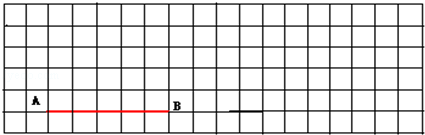首页 / 小学数学 / 试卷选题

# 苏教版五年级（上）期末数学

 1.4+3.6= 0.3×0.3= 0.49÷7= 7.2÷100= 3.6÷0.6= 1﹣0.8÷2= 1﹣0.7= 4.2÷3= 15×0.4= 1÷0.25= 0.23×0.5=

• 题型：未知
• 难度：未知

19﹣0.07=
0.63×3.6=
9.45+0.65=
5.15÷86≈（保留两位小数）

• 题型：未知
• 难度：未知

6.8+0.98+0.2
43.5+43.5×99
1.25×7.2
11÷[（0.4+0.04）×0.5]．

• 题型：未知
• 难度：未知

6.05吨=　　　　　　千克
2.4公顷=　　　　　　平方米
80公顷=　　　　　　平方千米
3.3厘米=　　　　　　米．

• 题型：未知
• 难度：未知• 题型：未知
• 难度：未知

• 题型：未知
• 难度：未知

• 题型：未知
• 难度：未知

• 题型：未知
• 难度：未知

• 题型：未知
• 难度：未知

• 题型：未知
• 难度：未知• 题型：未知
• 难度：未知

• 题型：未知
• 难度：未知

• 题型：未知
• 难度：未知

• 题型：未知
• 难度：未知

• 题型：未知
• 难度：未知

• 题型：未知
• 难度：未知

• 题型：未知
• 难度：未知

A．795÷1.3    B．79.5÷1.3   C．7950÷1.3

• 题型：未知
• 难度：未知

A．64÷16      B．0.64÷0.8   C．6.4÷0.08

• 题型：未知
• 难度：未知

A．变大 B．变小 C．不变

• 题型：未知
• 难度：未知

A．90个       B．99个 C．100个

• 题型：未知
• 难度：未知

A．1平方米  B．10平方米 C．1公顷

• 题型：未知
• 难度：未知• 题型：未知
• 难度：未知

• 题型：未知
• 难度：未知

• 题型：未知
• 难度：未知

• 题型：未知
• 难度：未知

• 题型：未知
• 难度：未知（1）　　　　　　月份两种果汁饮料销售数量的差距最大．
（2）甲品牌饮料平均每个月销售多少箱？

• 题型：未知
• 难度：未知

• 题型：未知
• 难度：未知